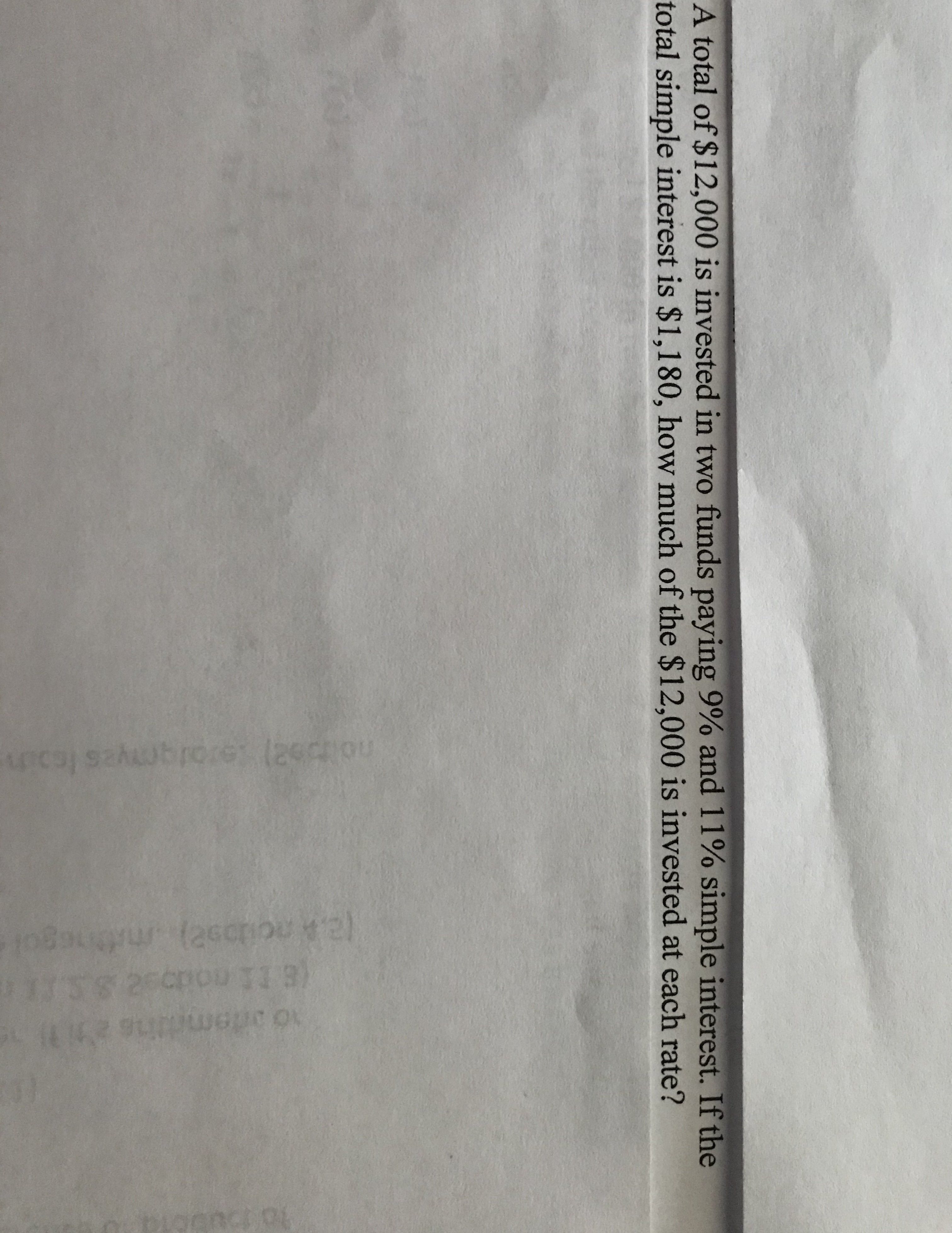# las(ascpou lotCEA total of \$12,000 is invested in two funds paying 9% and 11% simple interest. If thetotal simple interest is \$1,180, how much of the \$12,000 is invested at each rate?

Question
2 views

how do i solve this application ? step by step pleasehelp_outlineImage Transcriptioncloselas (ascpou l ot CE A total of \$12,000 is invested in two funds paying 9% and 11% simple interest. If the total simple interest is \$1,180, how much of the \$12,000 is invested at each rate? fullscreen
check_circle

Step 1

The total invested amo...

### Want to see the full answer?

See Solution

#### Want to see this answer and more?

Solutions are written by subject experts who are available 24/7. Questions are typically answered within 1 hour.*

See Solution
*Response times may vary by subject and question.
Tagged in

### Calculus